# Massive field

A quantum field theory contains massive fields if the Hilbert space obtained by repeated application of these fields to the vacuum carries a unitary representation of the covering group of the orthochronous proper Poincaré group (cf. also Poincaré group) which fulfills the mass-gap condition: Let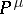(withreferring to the "time variable" ) be the generators of the space-time translations and let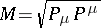be the mass operator.is well defined due to the positive-energy condition, i.e. the condition that the joint spectrum oflies in the forward lightcone [a1]. The mass-gap condition then says that the spectrum oflies in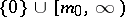, where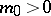and the multiplicity of the zero-eigenvector ofis one (uniqueness of the vacuum).

By the analysis of E. Wigner [a2], [a3], all states which describe a single particle form a Hilbert subspace carrying an irreducible representation of the Poincaré group which is labelled by a pair. Here,is the eigenvalue of these states with respect to the mass operatorand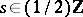, called the "spin" of the particle, labels the finite-dimensional representation of the little group stabilizing a vectorin the Minkowski space-time with,, i.e. the covering group of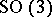. As a consequence of the mass gap assumption, all particles in a theory with massive fields have positive mass. Since one-particle states are usually assumed to be the states of lowest energy (above that of the vacuum), the mass-gap assumption and the assumption that a quantum field theory contains only particle states with positive mass, are considered as equivalent assumptions.

In the case that the one-particle states with the label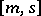are separated from the rest of the mass spectrum by a second mass-gap, i.e. the spectrum oflies in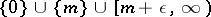for some, and there is some quantum field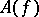in the theory such thatfor some Schwartz test function(cf. also Generalized functions, space of), with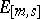the projector on the Hilbert subspace on the-one-particle states, one can apply the Haag–Ruelle scattering theory [a4], [a5], [a16] to: Letbe Schwartz test functions, such that the Fourier transform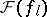of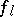has support in the set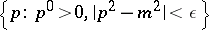. Setting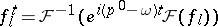, one defines asymptotic fields by their action on the vacuum vector: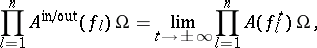where the vectors on the right-hand side converge in the strong Hilbert space topology. The asymptotic fieldsare free fields and generate a Fock space of multi-particle in- and out-states over the space of one-particle states with label. If these in- and out-Fock spaces span the whole Hilbert space of the theory (the so-called requirement of asymptotic completeness) then, as a corollary to the PCT theorem, the scattering matrix taking in-states to the related out-states is unitary [a6]. The requirements of Haag–Ruelle theory alone suffice to derive the LSZ-reduction formulas [a7], which express the scattering matrix elements (scalar product of in- and out-states, which gives the physical transition amplitude) via the time-ordered vacuum expectation values of the field[a8]. This links the general formalism of quantum fields [a1], [a6] to the heuristic perturbation expansions for the time-ordered Wightman functions based on the classical Lagrangian and the heuristic path integral (cf. also Quantum field theory).

From the 1960s onwards, a systematic construction of rigorous (non-perturbative) models has been started in space-time dimensions, see [a9], [a10], [a11]; for models witharbitrary (however with a state space carrying an indefinite inner product), see e.g. [a12].

Massive quantum field theory is taken to be an approximation to the real physical situation, where all long range forces, associated with massless fields, can be neglected as "weak" in comparison with the strong short range forces associated with massive fields. If only massive fields are present in a theory, the mathematical treatment of the theory is simpler, due to the absence of a number of effects connected with massless particles and fields (cf. Massless field). However, several features of the contemporary (2000) physical theory of strong interactions, as e.g. "quarks" , "confinement" and "asymptotic freeness" , are not yet well explained in the given mathematical framework (but see e.g. [a13] for an interesting new approach).

Massive classical fields are studied in the framework of non-linear hyperbolic partial differential equations (cf. also Hyperbolic partial differential equation), see e.g. [a14], [a15].